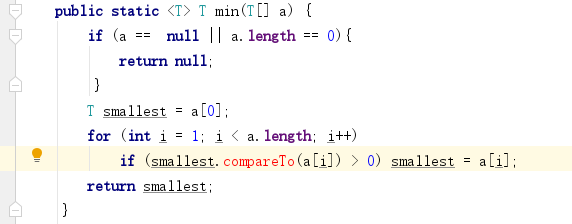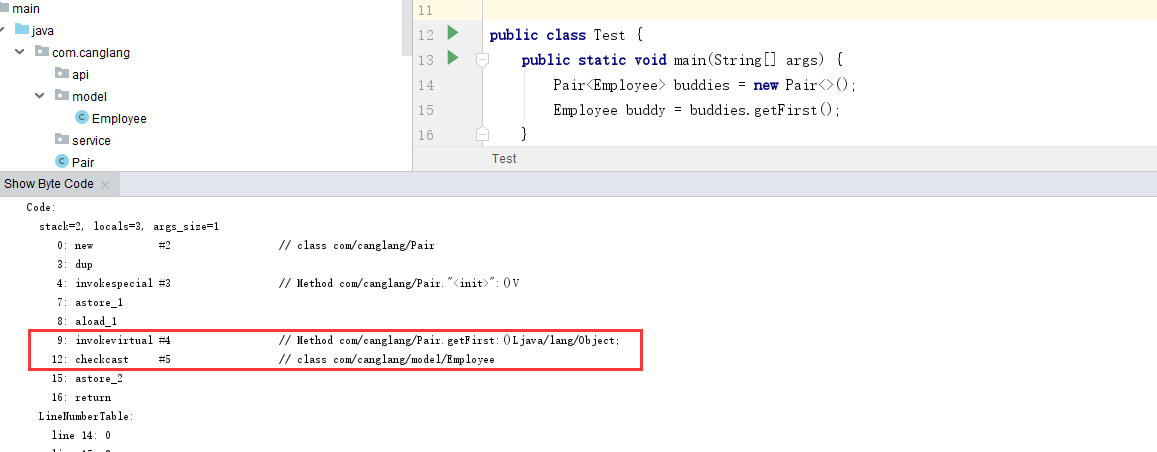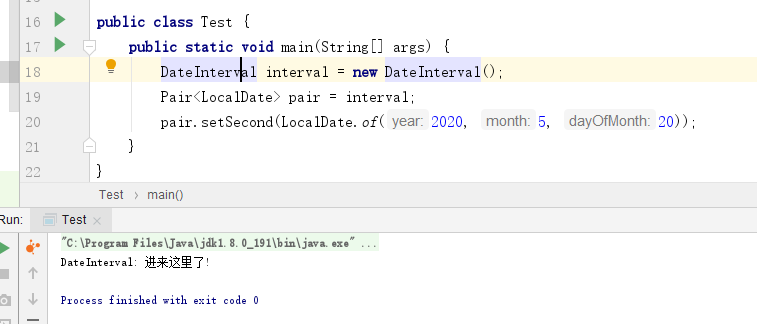# Java的泛型详解

## 泛型的好处

• 编写的代码可以被不同类型的对象所重用。
• 因为上面的一个优点，泛型也可以减少代码的编写。

## 泛型的使用

### 简单泛型类

``````public class Pair {

private  T first;

private T second;

public Pair() {
first = null;
second = null;
}

public Pair(T first, T second){
this.first = first;
this.second = second;
}

public T getFirst(){
return  first;
}

public T getSecond(){
return second;
}

public void setFirst(T first) {
this.first = first;
}

public void setSecond(T second) {
this.second = second;
}
}
``````
• 上面例子可以看出泛型变量为T;
• 用尖括号(<>)括起来,并放在类名后面;
• 泛型还可以定义多个类型变量比如上面的例子 first和second不同的类型:
` public class Pair {....}`

(1) 类型变量使用大写形式,并且要比较短;
(2)常见的类型变量特别代表一些意义:变量E 表示集合类型,K和V表示关键字和值的类型;T、U、S表示任意类型；

• 类定义的类型变量可以作为方法的返回类型或者局部变量的类型;

• 用具体的类型替换类型变量就可以实例化泛型类型;
` 例如: Pair 代表将上述所有的T 都替换成了String`
• 由此可见泛型类是可以看作普通类的工厂

### 泛型方法

• 我们应该如何定义一个泛型方法呢?
• 泛型的方法可以定义在泛型类,也可以定义在普通类,那如果定义在普通类需要有一个尖括号加类型来指定这个泛型方法具体的类型;
``````public class TestUtils {
public static  T getMiddle(T... a){
return  a[a.length / 2];
}
}
``````
• 类型变量放在修饰符(static)和返回类型的中间;
• 当你调用上面的方法的时候只需要在方法名前面的尖括号放入具体的类型即可;
``````String middle = TestUtils.getMiddle("a", "b", "c");
``````

``````String middle = TestUtils.getMiddle("a", "b", "c");
``````### 类型变量的限定

• 有时候我们不能无限制的让使用者传递任意的类型,我们需要对我们泛型的方法进行限定传递变量,比如如下例子• 这个时候是无法编译通过的,且编译器会报错
• 因为我们的编译器不能确定你这个T 类型是否有`compareTo`这个函数,所以这么能让编译器相信我们这个T是一定会有`compareTo`呢?
• 我们可以这么写 这里的意思是T一定是继承`Comparable`的类
• 因为`Comparable`是一定有`compareTo`这个方法,所以T一定有`compareTo`方法,于是编译器就不会报错了
• 因为加了限定那么`min`这个方法也只有继承了`Comparable`的类才可以调用;
• 如果要限定方法的泛型继承多个类可以加`extends` 关键字并用`&`分割如:`T extends Comparable & Serializable`
• 限定类型是用`&`分割的,逗号来分割多个类型变量

## 类型擦除

`````` public class Pair {

private  Object first;

private Object second;

public Pair() {
first = null;
second = null;
}

public Pair(Object first, Object second){
this.first = first;
this.second = second;
}

public Object getFirst(){
return  first;
}

public Object getSecond(){
return second;
}

public void setFirst(Object first) {
this.first = first;
}

public void setSecond(Object second) {
this.second = second;
}
}
``````
• 因为上面的T是没有限定变量,于是用Object代替了;
• 如果有限定变量则会以第一个限定变量替换为原始类型如:
``````public class Interval implements Serializable{
private T lower;
private T upper;
}
``````
• 原始类型如下所示:
``````public class Interval  implements Serializable{
private Comparable lower;
private Comparable upper;
}
``````

### 翻译泛型表达式

• 上面说到泛型擦除类型变量后对于无限定变量后会以Object来替换泛型类型变量;
• 但是我们使用的时候并不需要进行强制类型转换;
• 原因是编译器已经强制插入类型转换;

`````` Pair buddies = ...;
Employee buddy = buddies.getFirst();
``````
• 擦除getFirst的返回类型后将返回Object类型,但是编译器自动插入Employee的强制类型转换,编译器会把这个方法调用翻译为两条虚拟机指令;
• 对原始方法Pair.getFirst的调用
• 将返回的Object类型强制转换为Employee类型;`9: invokevirtual #4 // Method com/canglang/Pair.getFirst:()Ljava/lang/Object;`
`12: checkcast #5 // class com/canglang/model/Employee`

• invokevirtual：虚函数调用，调用对象的实例方法，根据对象的实际类型进行派发，支持多态;
• checkcast：用于检查类型强制转换是否可以进行。如果可以进行，checkcast指令不会改变操作数栈，否则它会抛出ClassCastException异常；

• 对于对象的实际类型进行替换泛型;
• 检查类型是否可以强制转换,如果可以将对返回的类型进行强制转换;

### 翻译泛型方法

``````public static  T min(T[] a)
``````

``````public static Comparable  min(Comparable[] a)
``````

``````public class DateInterval extends Pair {
public void setSecond(LocalDate second){
System.out.println("DateInterval: 进来这里了!");
}
}
``````

``````public void setSecond(Object second)
``````

``````public class Test {
public static void main(String[] args) {
DateInterval interval = new DateInterval();
Pair pair = interval;
pair.setSecond(LocalDate.of(2020, 5, 20));
}
}
``````

Pair引用了DateInterval对象,所以应该调用DateInterval.setSecond。17: invokestatic #4 // Method java/time/LocalDate.of:(III)Ljava/time/LocalDate;
20: invokevirtual #5 // Method com/canglang/Pair.setSecond:(Ljava/lang/Object;)V

Java编译器生成了一个桥接方法，以确保子类型能够按预期工作。

``````public class DateInterval extends Pair {
// Bridge method generated by the compiler
//
public void setSecond(Object second) {
setSecond((LocalDate)second);
}
public void setSecond(LocalDate second){
System.out.println("DateInterval: 进来这里了!");
}
}
``````

public void setSecond(java.lang.Object);
descriptor: (Ljava/lang/Object;)V
flags: ACC_PUBLIC, ACC_BRIDGE, ACC_SYNTHETIC
Code:
stack=2, locals=2, args_size=2